# Forecasting Calculators

The 5 Forecasting Calculators are found under the Forecasting tab. These are used to perform performance calculations using the estimates provided. Each Calculator has 11 different valuation lines with clickable points for each of the four quarters in the fiscal year.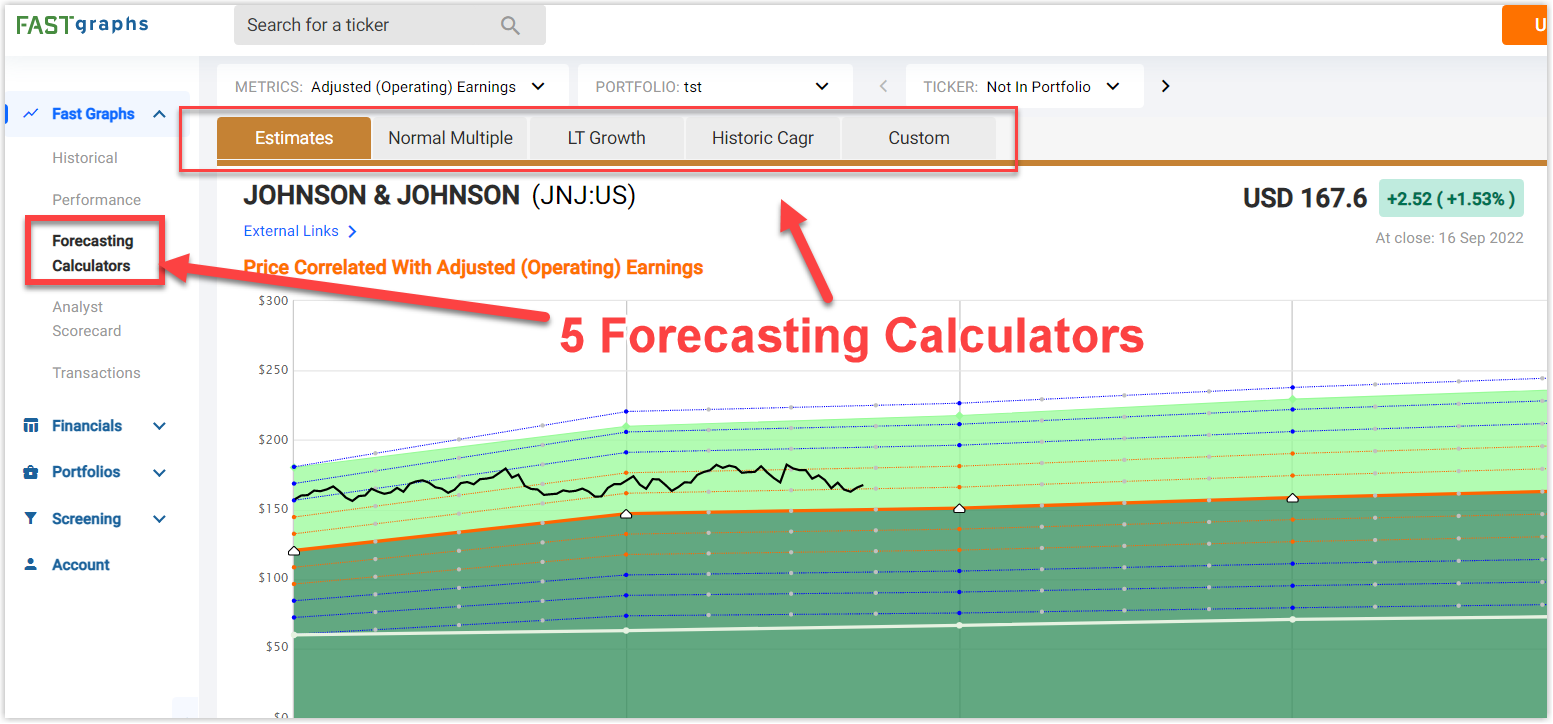The 5 Forecasting Calculators include:

• Estimates
• Normal Multiple
• LT Growth
• Historical CAGR
• Custom

## Estimates Calculator

The "Estimates" Calculator is the default calculator when going to the Forecasting tab. This can show up to 3 years of estimates and are the same estimates found on the Historical Graph.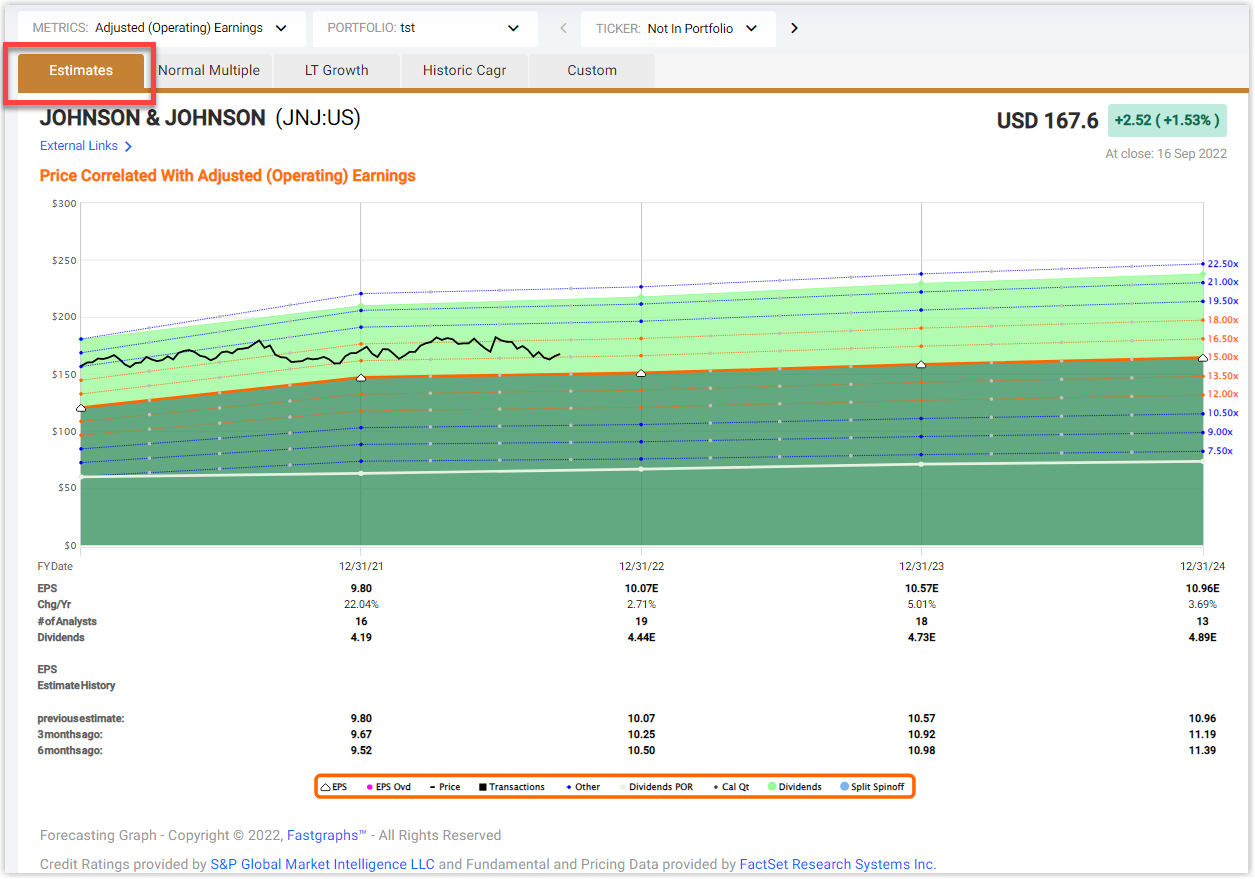The fair valuation line is drawn as the thick solid orange line, and its multiple depends on the estimated growth of the selected metric. The other calculation lines are drawn around it from the same estimates but with different multiples. These multiples can be seen on the right side of the graph.

The numbers below the graph give more insight into the data. Here you will find:

• The last actual number followed by the estimates for the selected metric
• The percent change per year
• The number of analysts making estimates for that specific year (This is extremely important to look at, because the more analysts there are for an estimate, the more reliable it can be)
• The last actual dividend followed by the dividend estimates
• The previous estimates
NOTE: This helps identify trends in analysts’ estimates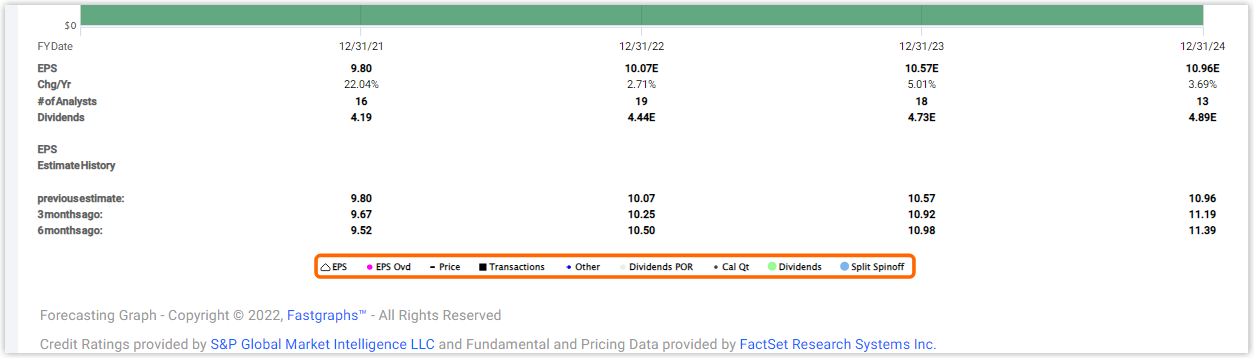## Normal Multiple Calculator

The "Normal Multiple" is the second calculator found under the Forecasting tab. This can show up to 3 years of estimates and are the same estimates found on the Historical Graph.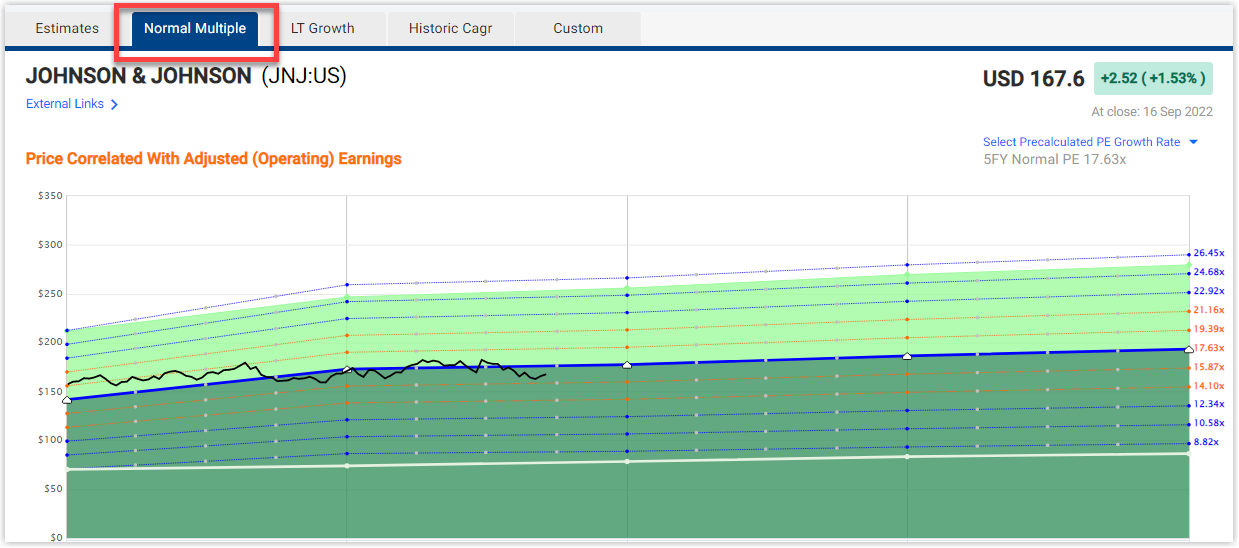The fair valuation line is drawn as the Normal PE applied to the Historical Graph and is represented as the thick solid blue line. The "Normal Multiple" Calculator defaults using a 5-year historical Normal PE. This can be changed by using the drop-down below the graph.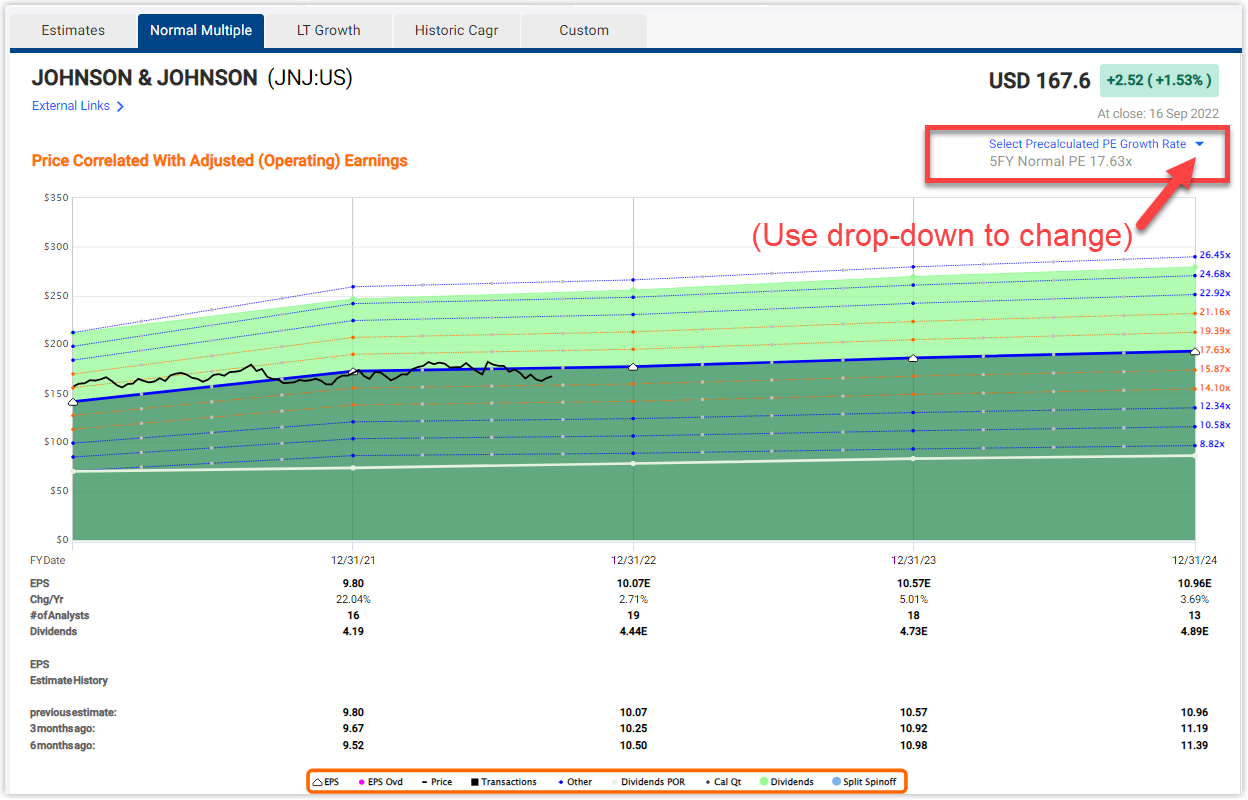The other calculation lines are drawn around it from the same estimates but with different multiples. These multiples can be seen on the right side of the graph.

## LT Growth Calculator

The "LT Growth" Calculator is the third forecasting calculator found under the Forecasting tab. This can show up to 5 years of trendline growth estimates from analysts.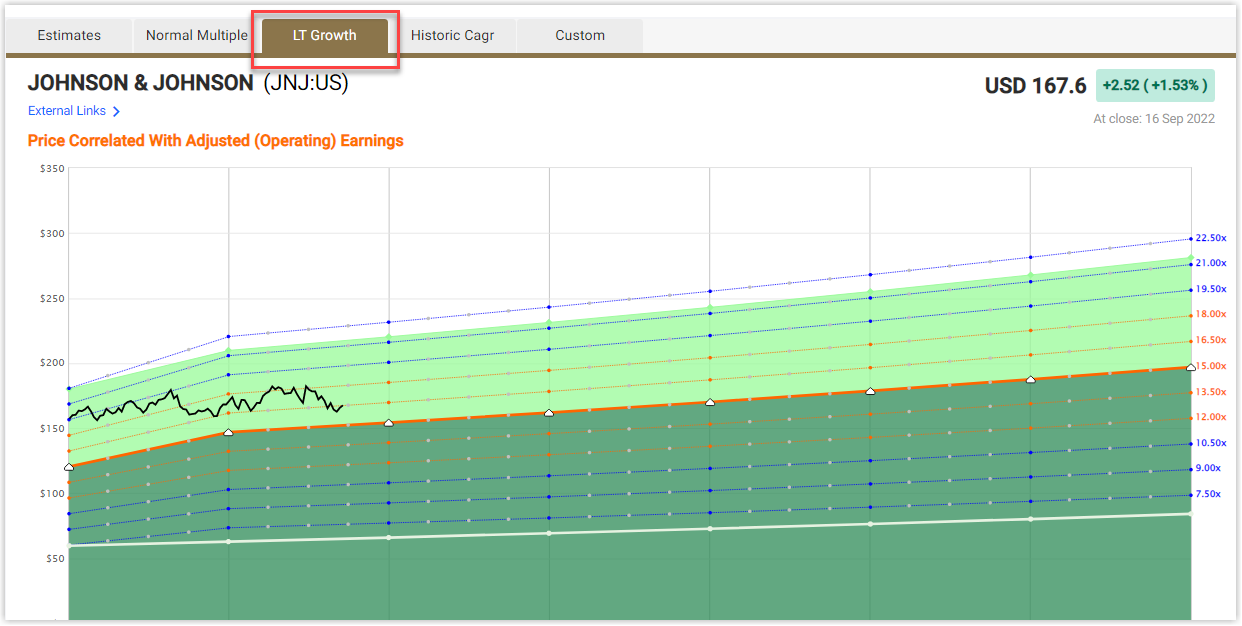The fair valuation line is drawn as the thick solid orange line, and its multiple depends on the estimated trendline growth of the selected metric. The other calculation lines are drawn around it from the same estimates but with different multiples. These multiples can be seen on the right side of the graph.

The numbers below the graph give more insight into the data. Here you will find:

• The last actual number followed by the estimates for the selected metric
These per share estimate numbers are calculated based on the estimated trendline growth rate
• The percent change per year
This will show the same across the bottom because this graph shows a trendline growth estimate
• The number of analysts making estimates for that specific year
• The last actual dividend followed by the dividend estimates grown at the LT growth rate

## Historical CAGR Calculator

The "Historical Compound Annual Growth Rate" Calculator is the fourth forecasting calculator found under the Forecasting tab. This can show up to 5 years of estimates calculated by applying a Historical Compound Annual Growth Rate (CAGR) from the company.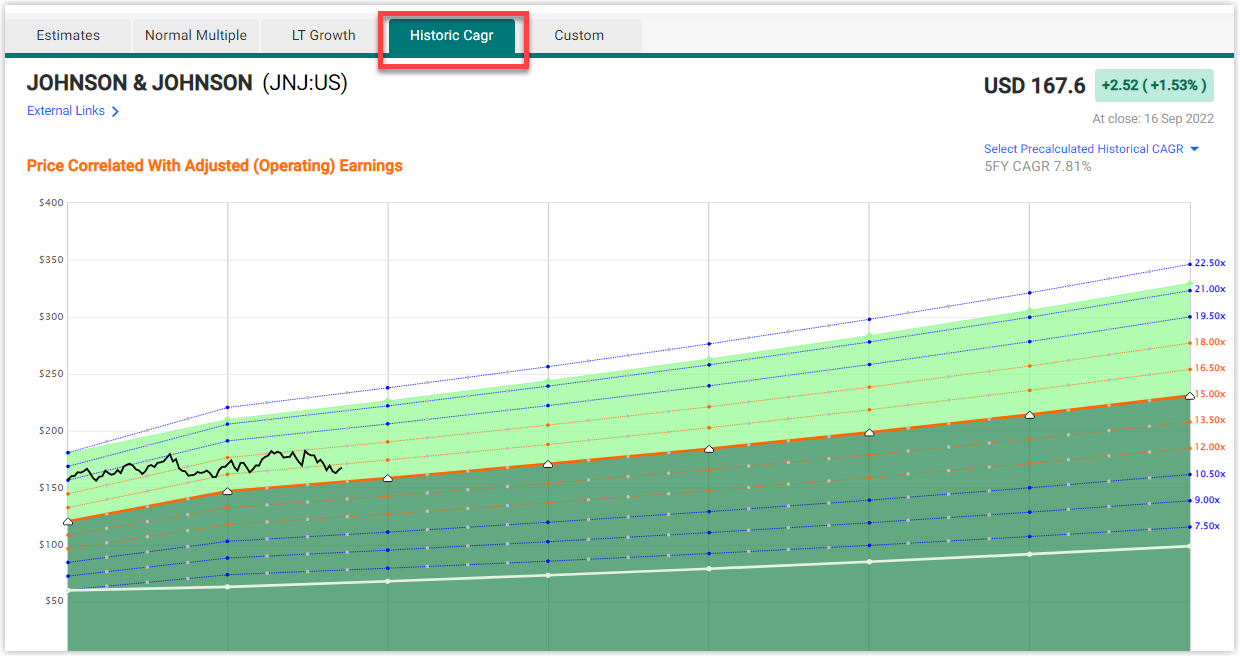The fair valuation line is drawn as the thick solid orange line, and its multiple depends on the selected Historical CAGR. The "Historical CAGR" Calculator defaults using a 5-year Historical CAGR. This can be changed by using the drop-down below the graph. The other calculation lines are drawn around it from the same estimates calculated from the selected Historical CAGR but with different multiples. These multiples can be seen on the right side of the graph.

The numbers below the graph give more insight on the data. Here you will find:

• The last actual number followed by the estimates for the selected metric
These per share estimate numbers are calculated based off of the selected Historical CAGR
• The percent change per year
This will show the same across the bottom because this graph applies the same Historical CAGR to each fiscal year
estimate
• The number of analysts making estimates for that specific year
This will always show N/A because there are no analysts estimating these estimates. These estimates are calculated
using the selected Historical CAGR
• The last actual dividend followed by the dividend estimates

## Custom Calculator

The "Custom" Forecasting Calculator is the fifth and final calculator available on FAST Graphs. It gives you the ability to enter your own numbers to experiment with your own scenarios. Any boxes with gray numbers that you find around the calculator can be changed to whatever value you want.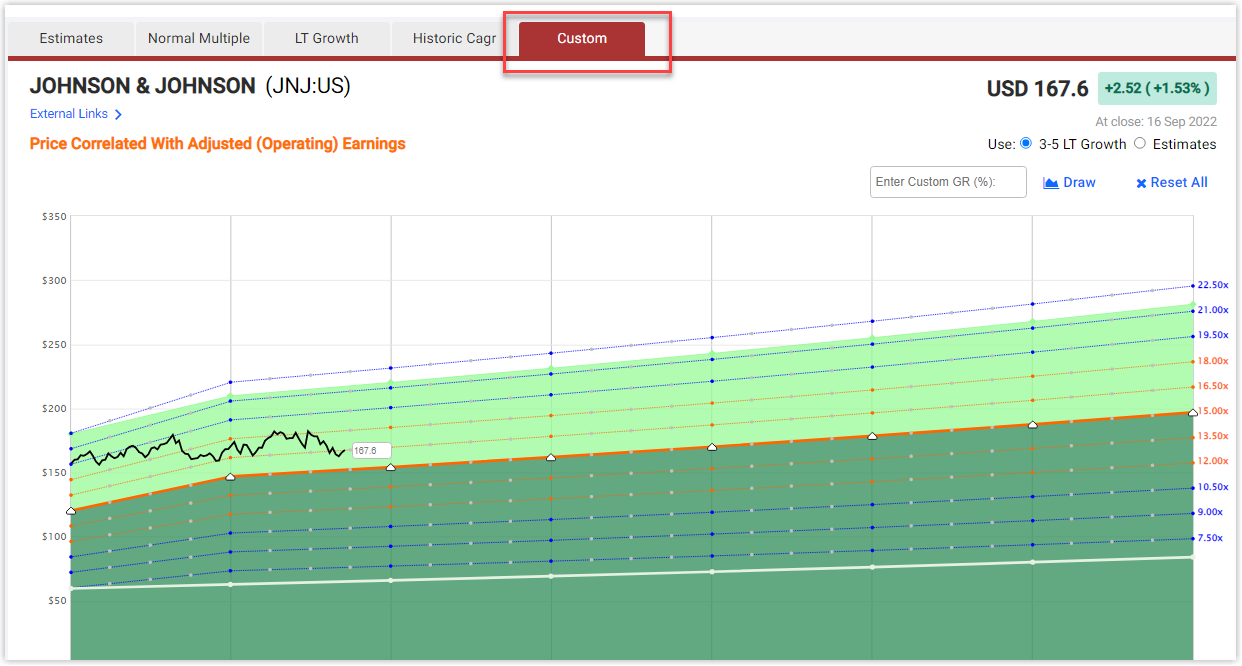This calculator extends out to 5 years and uses trendline estimates as its default. You can also blend the 3-5 Year Trendline estimates with the individual Estimates giving you a different picture. The power of this is looking at individual estimates for up to 3 years, and then extending the estimates out to 5 years using the estimated trendline growth. This is done by using the select boxes underneath the graph.

A custom valuation multiple can also be added to the calculator. This can be changed in the box to the right of the graph, where all of the multiples are displayed.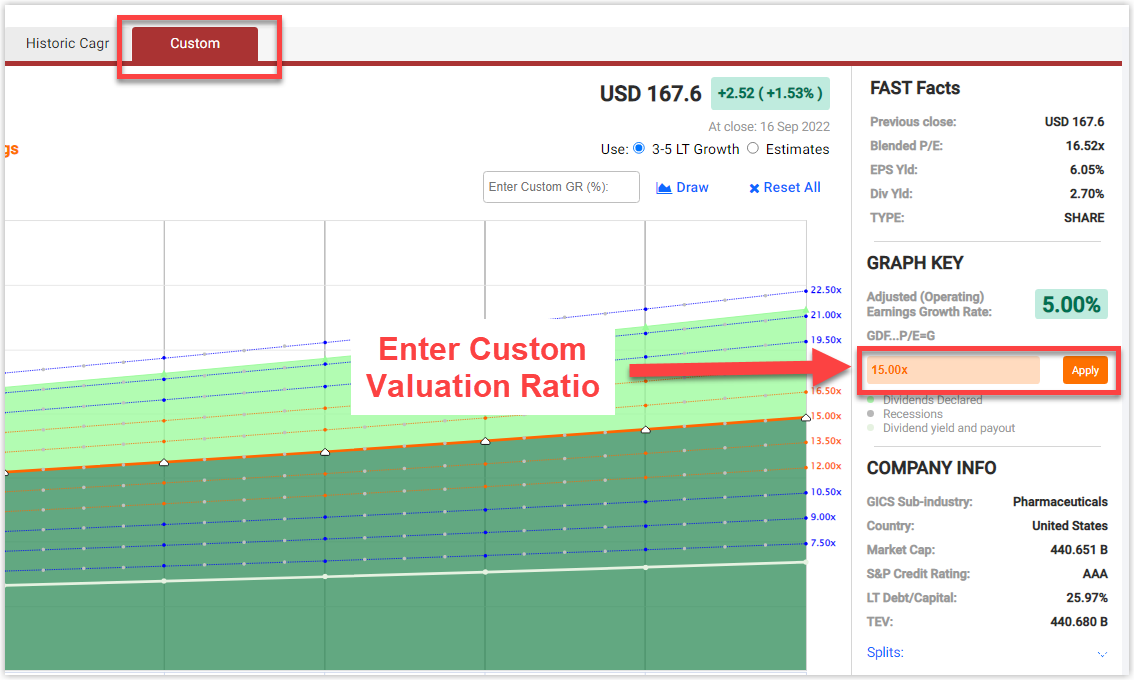Most of the numbers below the calculator can be changed too, giving you the ability to input your own custom estimates and dividend estimates to further customize your analysis.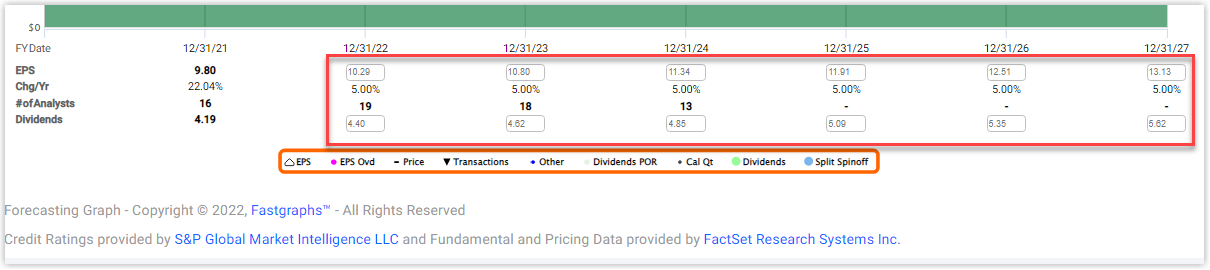The Custom Calculator allows you to input your own custom growth rate so that you can experiment with different rates at which the selected metric may grow.

To reset everything back to default, click the "RESET ALL" button.